• 尽管二叉树节点中存储的数据类型是任意的，但为了大小比较和理解的方便，本文中将数据类型指定为整数。
• 各个节点中的存储的数据是没有重复的。

## 定义

• 对于树中的任意节点 X，若其左子树不为空，则左子树中每一个节点的值都小于其根节点的值
• 对于树中的任意节点 X，若其右子树不为空，则右子树中每一个节点的值都大于或等于其根节点的值
• 对于树中的任意节点 X，其左子树、右子树也为二叉查找树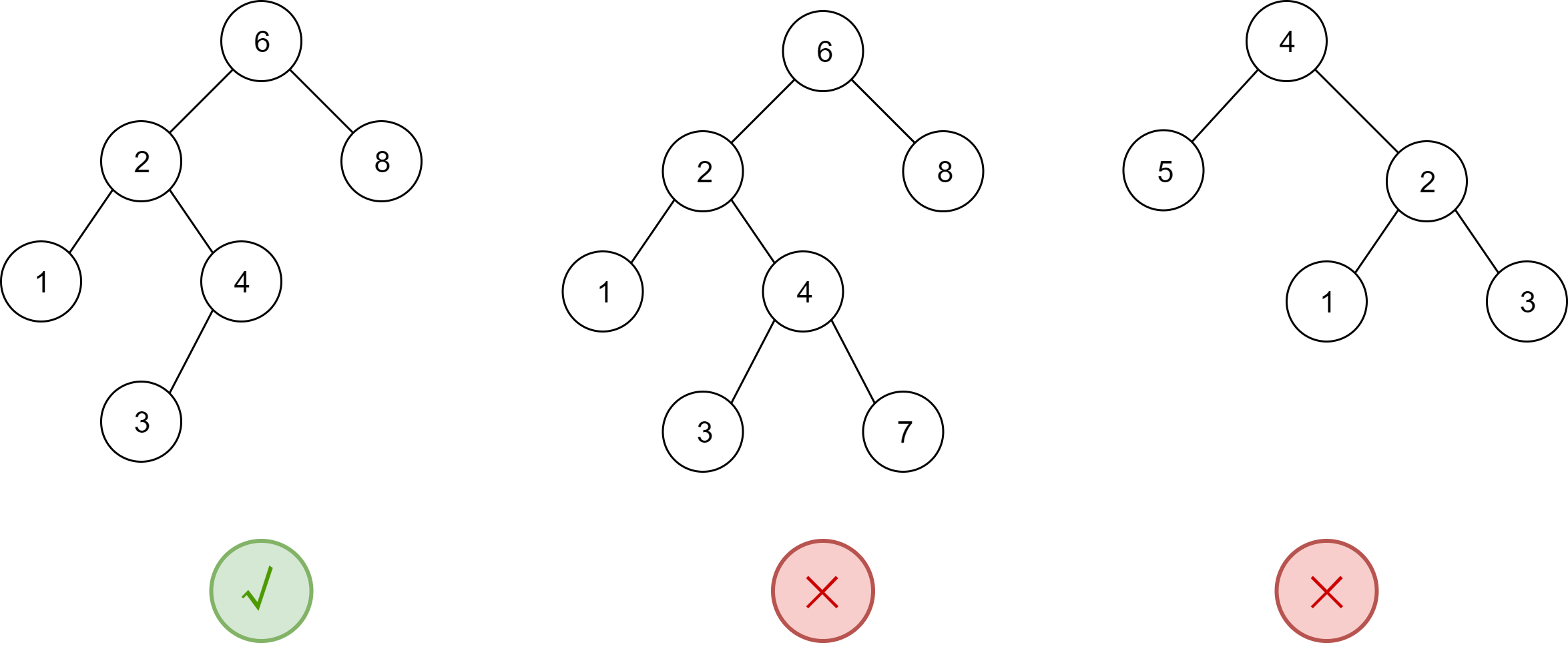## 声明

typedef int ElementType;

struct TreeNode;
typedef struct TreeNode *Position;
typedef struct TreeNode *SearchTree;

/*BST 的基本操作定义 */
SearchTree MakeEmpty(SearchTree T);
Position Find(ElementType X, SearchTree T);
Position FindMin(SearchTree T);
Position FindMax(SearchTree T);
SearchTree Insert(ElementType X, SearchTree T);
SearchTree Delete(ElementType X, SearchTree T);
ElementType Retrieve(Position P);

/* 单个树节点的结构体定义 */
struct TreeNode
{
ElementType Element;
SearchTree Left;
SearchTree Right;
};


## 基本操作

### 初始化 MakeEmpty

/* 初始化一棵树 T*/
SearchTree MakeEmpty(SearchTree T)
{
if (T != NULL)
{
MakeEmpty(T->Left);
MakeEmpty(T->Right);
free(T);
}
return NULL;
}


### 查找任意值 Find

/* 对树 T 查找值 X 的位置 */
Position Find(ElementType X, SearchTree T)
{
if (T == NULL)
return NULL;
if (X < T->Element)
return Find(X, T->Left);
else if (X> T->Element)
return Find(X, T->Right);
else
return T;
}


### 查找最小/最大值 FindMin/FindMax

/*FindMin 操作的递归实现 */
Position FindMin(SearchTree T)
{
if(T == NULL)
return NULL;
else
if(T->Left == NULL)
return T;
else
return FindMin(T->Left);
}


/*FindMin 操作的非递归实现 */
Position FindMin(SearchTree T)
{
if(T != NULL)
while(T->Left != NULL)
T = T->Left;

return T;
}


### 插入 Insert

/* 将值 X 插入到二叉查找树 T 中 */
SearchTree Insert(ElementType X, SearchTree T)
{
if (T == NULL)
{
T = malloc(sizeof(struct TreeNode));
if (T == NULL)
return NULL; /*Out of space*/
else
{
T->Element = X;
T->Left = T->Right = NULL;
}
}
else if (X < T->Element)
T->Left = Insert(X, T->Left);
else if (X> T->Element)
T->Right = Insert(X, T->Right);

return T;
}


int i;
int a = {67, 73, 129, 25, 101, 310, 123};
SearchTree *T = NULL;
for (i = 0; i < 7; i++)
{
Insert(a[i], T);
}


### 删除 Delete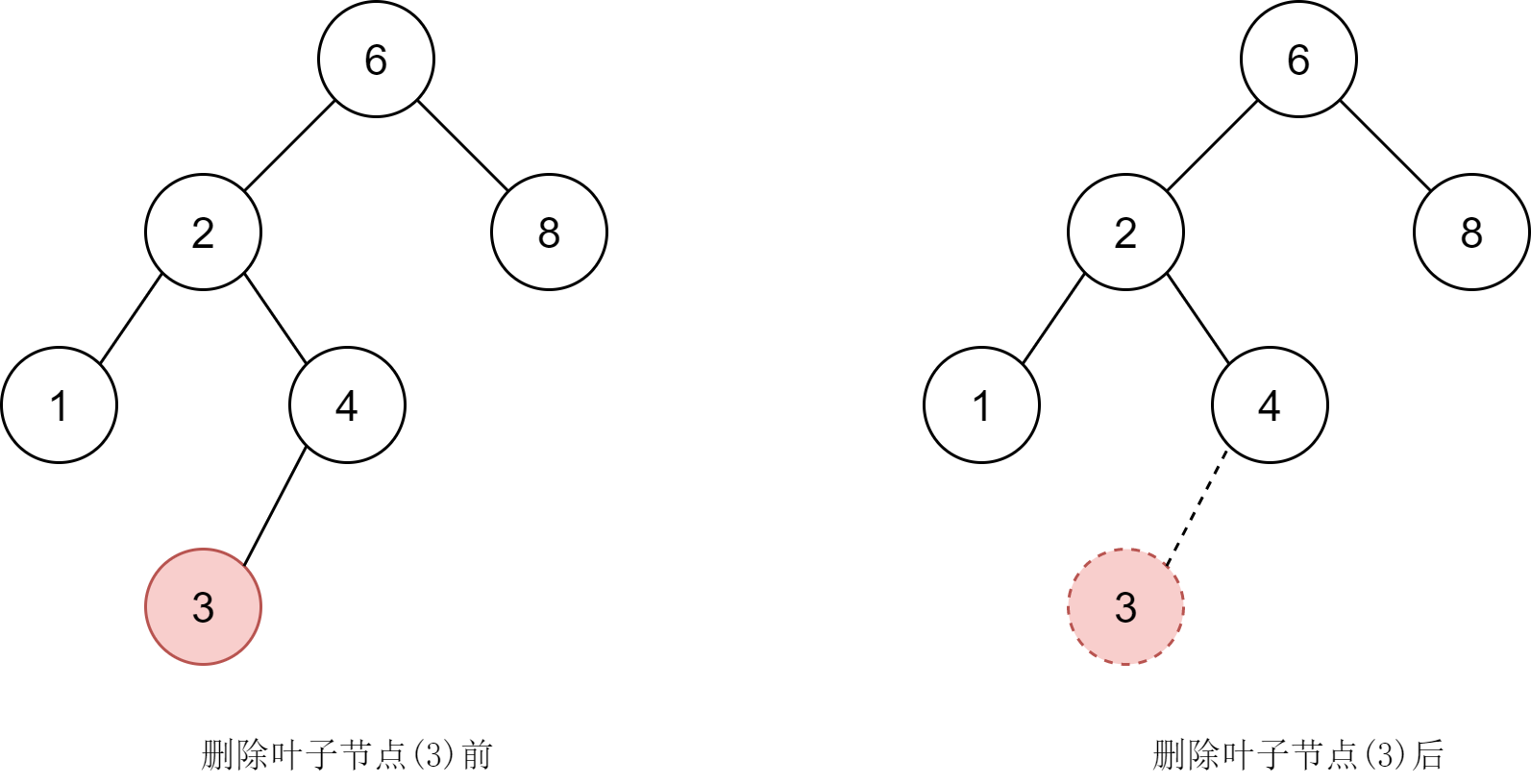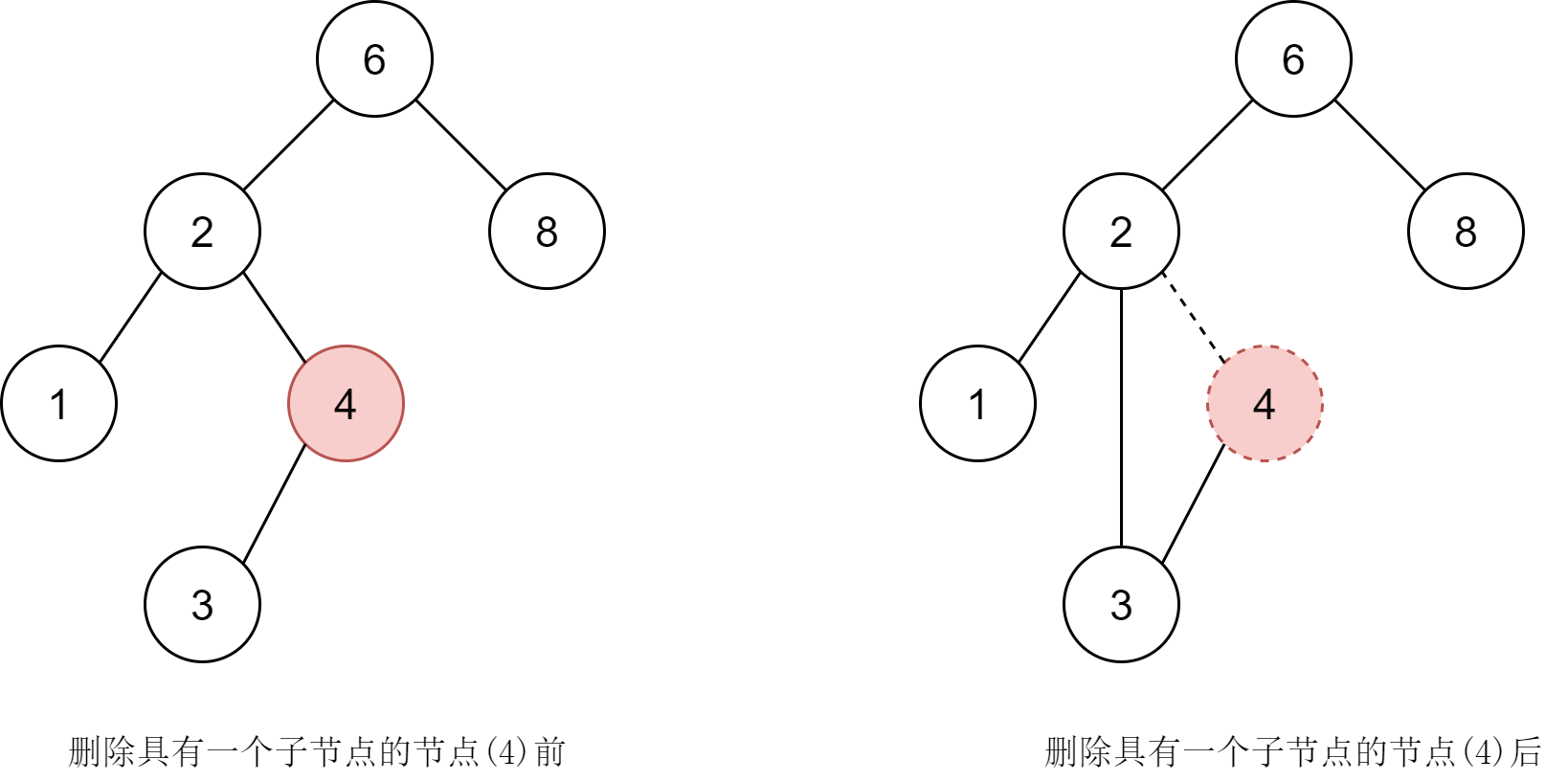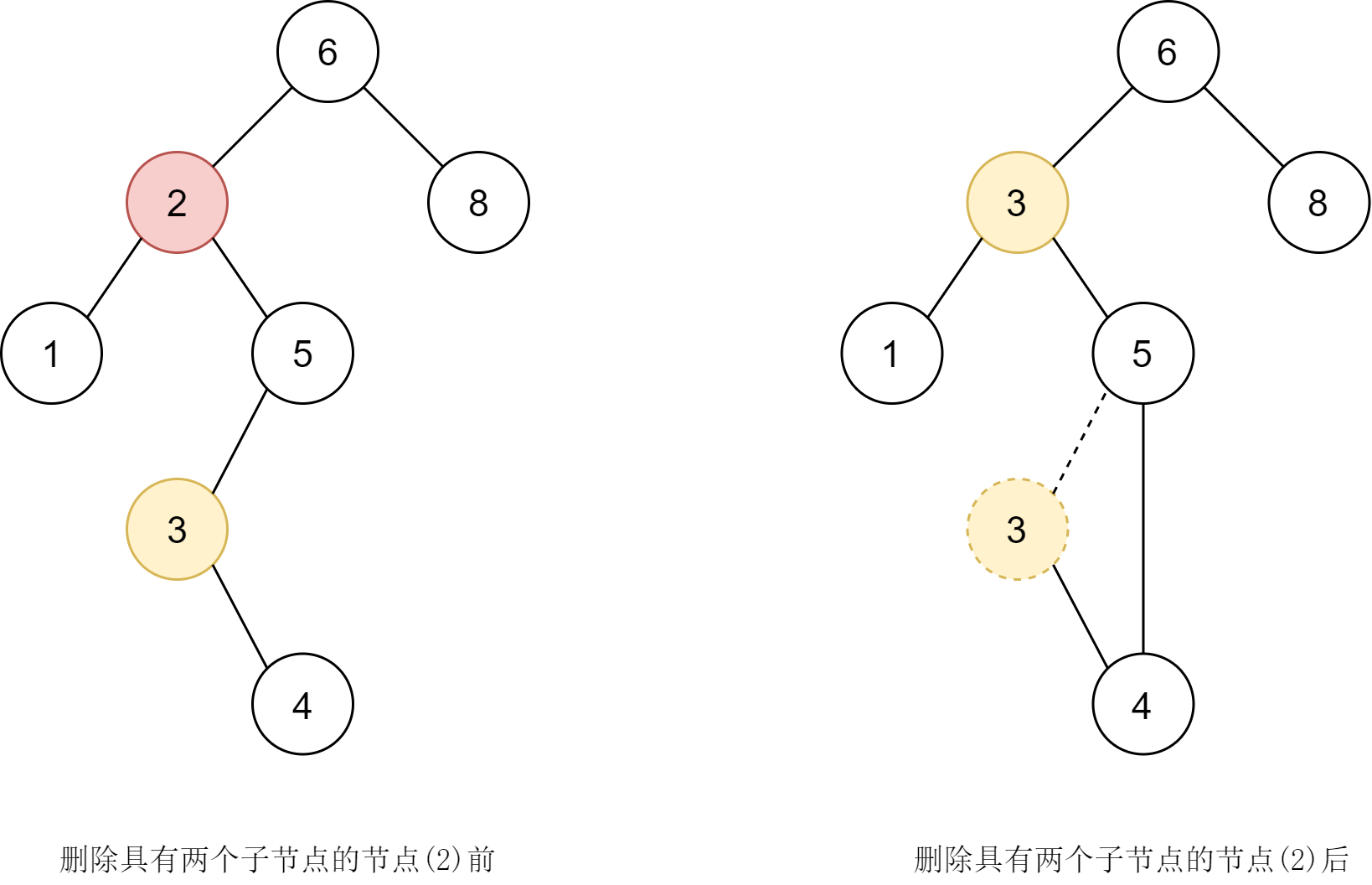/* 从树 T 中删除值为 X 的节点 */
SearchTree Delete(ElementType X, SearchTree T)
{
Position TmpCell;

if (T == NULL)
else if (X < T->Element)
T->Left = Delete(X, T->Left);
else if (X> T->Element)
T->Right = Delete(X, T->Right);
else
if (T->Left && T->Right)
{
TmpCell = FindMin(T->Right);
T->Element = TmpCell->Element;
T->Right = Delete(T->Element, T->Right);
}
else
{
TmpCell = T;
if (T->Left == NULL)
T = T->Right;
else if (T->Right == NULL)
T = T->Left;
free(TmpCell);
}
return T;
}


## 性能分析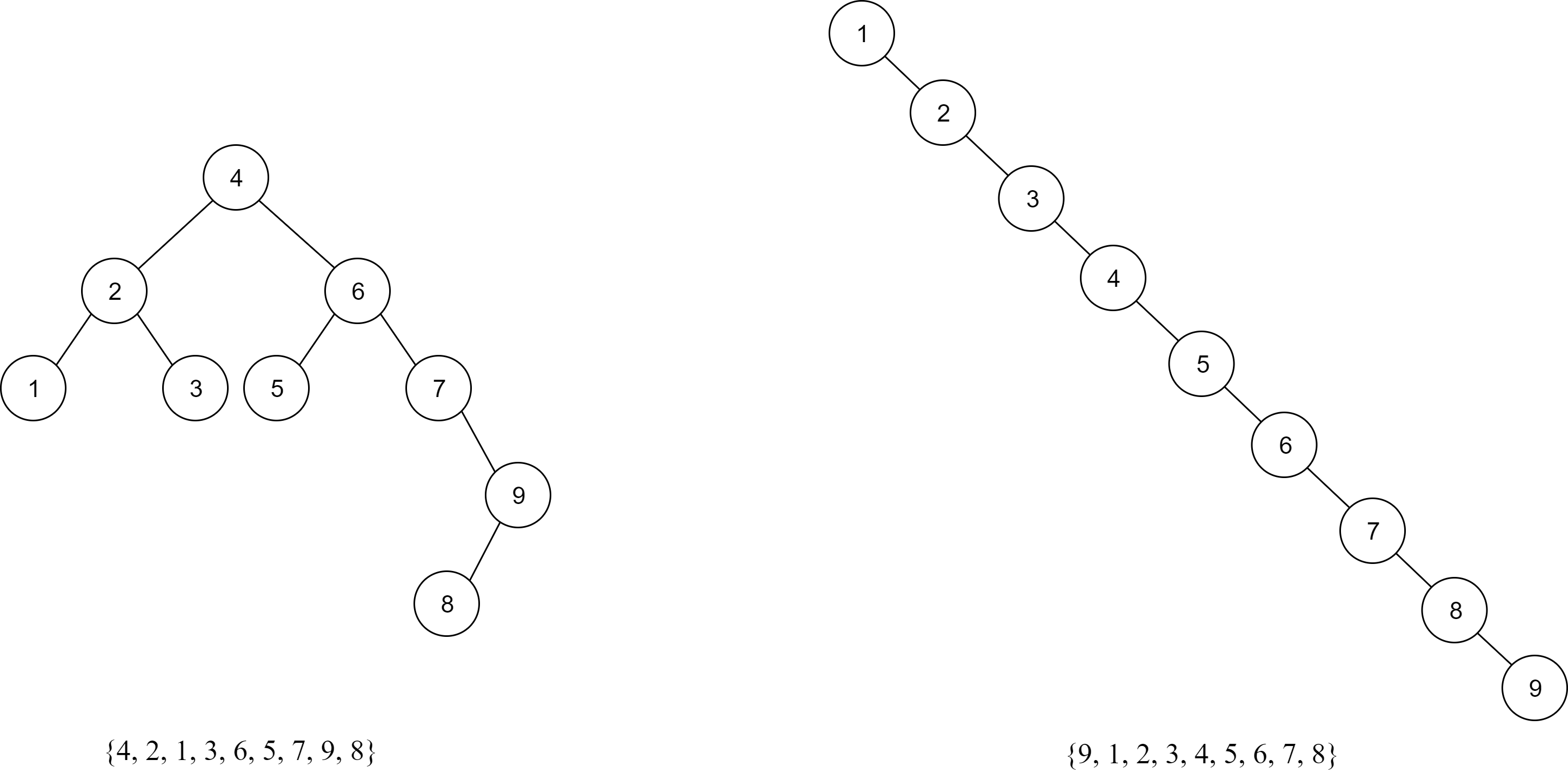## 参考资料

 (美)Mark Allen Weiss. 数据结构与算法分析: C 语言描述 [M]. 机械工业出版社: 北京, 2017:73-80.
 程杰. 大话数据结构 [M]. 清华大学出版社: 北京, 2011:313-328.
 维基百科：二叉搜索树 [EB/OL].https://zh.wikipedia.org/wiki/二叉搜索树,2020-2-14.